O 1 O O 1 O O 1 O 1 1 O 1 1 O 1 O 1 1 O O O O 1 O 1 1 O O 1 1 1 O 1 1 O 1 O O 1 O 1 1 O 1 1 1 O O 1 1 O O O O 1 O 1 1 1 O 1 O O O 1 1 O 1 O O 1 O 1 1 O 1 1 1 1 O 1 1 O 1 1 1 O O O 1 O O O O O O 1 1 O 1 O O 1 O 1 1 1 O O 1 1 O O 1 O O O O O O 1 1 O 1 1 O 1 O 1 1 O 1 1 1 1 O 1 1 1 O O 1 O O 1 1 O O 1 O 1 O O 1 O O O O O O 1 1 O 1 O O 1 O 1 1 O 1 1 O 1 O 1 1 1 O O O O O 1 1 O 1 1 1 1 O 1 1 1 O O 1 O O 1 1 1 O 1 O O O 1 1 O O O O 1 O 1 1 O 1 1 1 O O 1 1 1 O 1 O O O O 1 O O O O O O 1 1 1 O 1 O O O 1 1 O 1 O O O O 1 1 O O O O 1 O 1 1 O 1 1 1 O O O 1 O O O O O O 1 1 O 1 O 1 1 O 1 1 O 1 1 1 O O 1 1 O 1 1 1 1 O 1 1 1 O 1 1 1 O 1 1 O 1 1 O O O 1 1 O O 1 O 1 O 1 1 O O 1 O O O 1 1 O O 1 1 1 O 1 1 O O 1 O 1 O O 1 O 1 1 1 O O O 1 O O O O O O 1 O O O 1 1 O O 1 1 O 1 1 1 1 O 1 1 1 O O 1 O O O 1 O O O O O O 1 1 O 1 O 1 1 O 1 1 O 1 1 1 O O 1 1 O 1 1 1 1 O 1 1 1 O 1 1 1 O 1 1 O 1 1 O O O 1 1 O O 1 O 1 O 1 1 O O 1 O O O 1 1 O O 1 1 1 O 1 1 O O 1 O 1 O O 1 O O O O O O 1 1 O 1 O O 1 O 1 1 1 O O 1 1 O O 1 O O O O O O 1 1 O 1 1 O O O 1 1 O 1 O O 1 O 1 1 O 1 1 O 1 O 1 1 O 1 O O 1 O 1 1 1 O 1 O O O 1 1 O O 1 O 1 O 1 1 O O 1 O O O O 1 O O O O O O 1 1 1 O 1 O O O 1 1 O 1 1 1 1 O O 1 O O O O O O 1 1 O O O O 1 O 1 1 O 1 1 O O O 1 1 O 1 1 O O O O 1 O O O O O O 1 1 1 O 1 1 1 O 1 1 O O 1 O 1 O O 1 O O O O O O 1 1 O 1 1 1 O O 1 1 O 1 1 1 1 O 1 1 1 O 1 1 1 O O 1 O O O O O O 1 1 O 1 O 1 1 O 1 1 O 1 1 1 O O 1 1 O 1 1 1 1 O 1 1 1 O 1 1 1 O O 1 O O O O O O 1 1 O O O O 1 O 1 1 O 1 1 1 O O 1 1 O O 1 O O O O 1 O O O O O O 1 1 1 O 1 O 1 O 1 1 O 1 1 1 O O 1 1 O O 1 O O O 1 1 O O 1 O 1 O 1 1 1 O O 1 O O 1 1 1 O O 1 1 O 1 1 1 O 1 O O O 1 1 O O O O 1 O 1 1 O 1 1 1 O O 1 1 O O 1 O O O O 1 O 1 1 O O O O 1 O O O O O O 1 1 1 O 1 1 1 O 1 1 O 1 O O O O 1 1 O 1 O O 1 O 1 1 O 1 1 O O O 1 1 O O 1 O 1 O O 1 O O O O O O 1 1 O 1 O O 1 O 1 1 O 1 1 O 1 O 1 1 O O O O 1 O 1 1 O O 1 1 1 O 1 1 O 1 O O 1 O 1 1 O 1 1 1 O O 1 1 O O O O 1 O 1 1 1 O 1 O O O 1 1 O 1 O O 1 O 1 1 O 1 1 1 1 O 1 1 O 1 1 1 O O O 1 O O O O O O 1 1 O O 1 O 1 O 1 1 O 1 1 O 1 O 1 1 O O O 1 O O 1 1 1 O O 1 O O 1 1 O O O O 1 O 1 1 O O O 1 1 O 1 1 O O 1 O 1 O 1 1 1 O O 1 1 O O 1 O O O O O O 1 1 1 O 1 O O O 1 1 O 1 O O O O 1 1 O O 1 O 1 O O 1 O O O O O O 1 1 O O 1 O 1 O 1 1 O 1 1 1 O O 1 1 1 O 1 O O O 1 1 O 1 O O 1 O 1 1 1 O O 1 O O 1 1 O O 1 O 1 O O 1 O O O O O O 1 1 1 O 1 1 1 O 1 1 O 1 1 1 1 O 1 1 1 O O 1 O O 1 1 O 1 1 O O O 1 1 O O 1 O O O O 1 O 1 1 O O O O 1 O O O O O O 1 1 O O O O 1 O 1 1 O 1 1 1 O O 1 1 O O 1 O O O O 1 O O O O O O 1 1 O O O O 1 O 1 1 O 1 1 O O O 1 1 O 1 1 O O O O 1 O O O O O O 1 1 1 O 1 O O O 1 1 O 1 O O O O 1 1 O O 1 O 1 O 1 1 1 O O 1 O O 1 1 O O 1 O 1 O O 1 O O O O O O 1 1 O O 1 O 1 O 1 1 1 O 1 1 O O 1 1 O O 1 O 1 O 1 1 1 O O 1 O O O 1 O O O O O O 1 1 1 O 1 1 1 O 1 1 O 1 O O 1 O 1 1 O 1 1 O O O 1 1 O 1 1 O O O O 1 O O O O O O 1 1 O O O 1 O O 1 1 O O 1 O 1 O O 1 O O O O O O 1 1 1 O 1 O O O 1 1 O 1 1 1 1 O O 1 O O O O O O 1 1 O 1 O 1 1 O 1 1 O 1 1 1 O O 1 1 O 1 1 1 1 O 1 1 1 O 1 1 1 O O 1 O O O O O O 1 1 O O O O 1 O 1 1 O 1 1 1 O O 1 1 O O 1 O O O O 1 O O O O O O 1 1 1 O 1 O 1 O 1 1 O 1 1 1 O O 1 1 O O 1 O O O 1 1 O O 1 O 1 O 1 1 1 O O 1 O O 1 1 1 O O 1 1 O 1 1 1 O 1 O O O 1 1 O O O O 1 O 1 1 O 1 1 1 O O 1 1 O O 1 O O
Video Rotate & Flip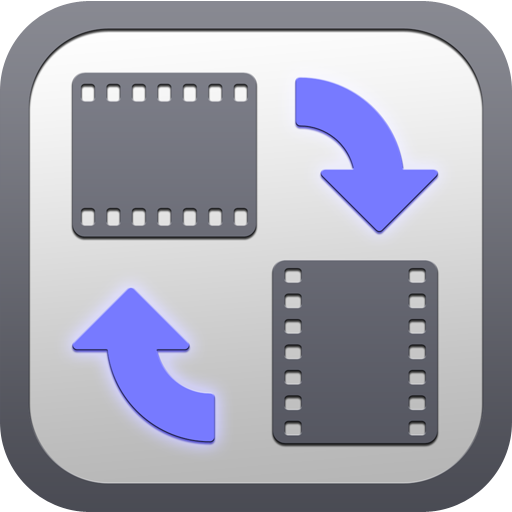# 提示

Video Rotate & Flipで、あなたのデバイスから直接、簡単で楽しく動画の回転や反転ができます。

Video Rotate & Flipはこんな場合に使えます:

• デバイスの回転ロックを解除し忘れて、良くない向きで動画を撮影してしまった。 Video Rotate & Flip を使って向きを変えましょう。
• 地面あるいは空の動画を撮った。デバイスが選択した向きが最適ではなく、変えたいと思っている。
• ミラー効果を入れて動画を左右反転させ、物事を読めなくしたい。
• 動画を上下逆にしたり、回転したり、ただおもしろい効果を入れたい。
• コンピューターの動画プレーヤーに、動画を正しく回転させないものがある。Video Rotate & Flipを使って動画の向きを修正しましょう。
ビデオがまだコンピュータ上読み取れない場合は、お使いのビデオプレイヤーは完全に。movファイル拡張子をサポートしないかもしれません。代わりにQuicktimeを使用してください。

これらのアプリケーションは、Appleやさまざまなウェブサイトで何回も取り上げられています。

ポートレートや風景対応。

# ビデオ

 (v1.5 on iPhone 6) (v1.5 on iPad)

# iPhoneスクリーンショット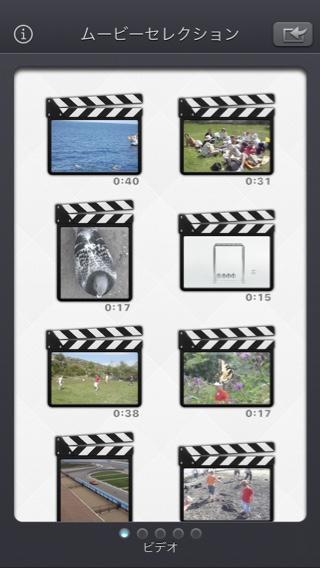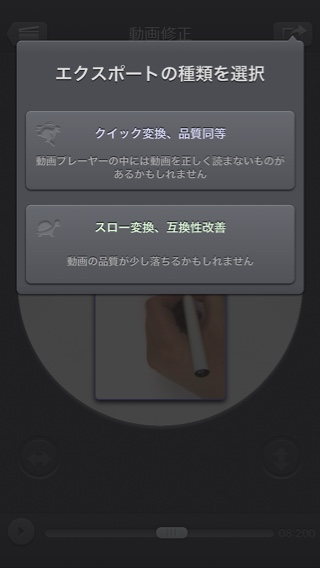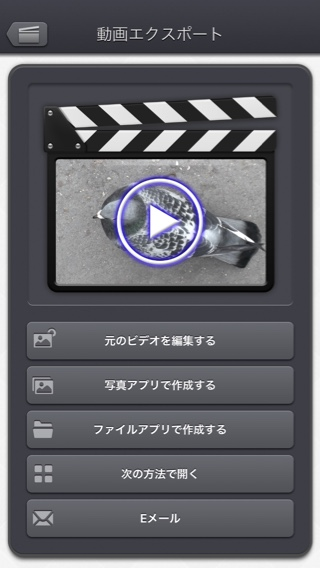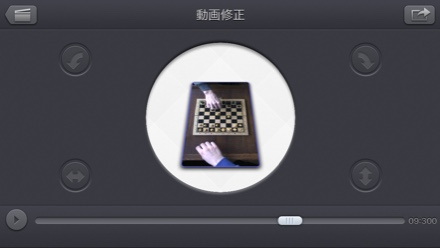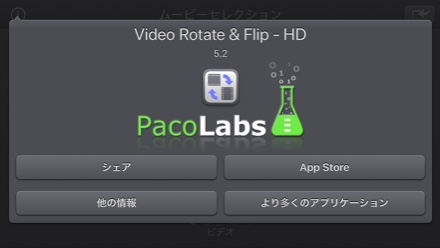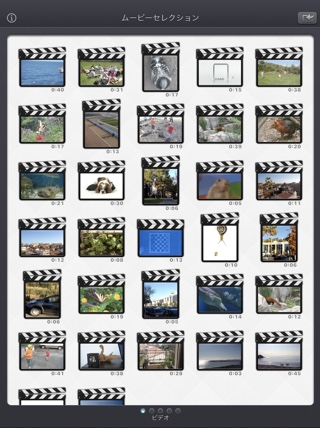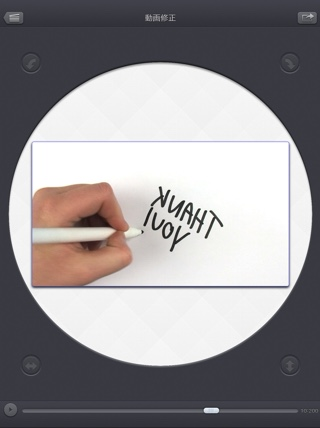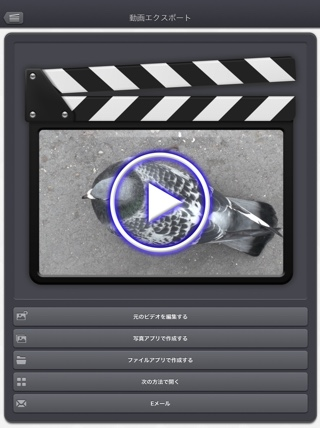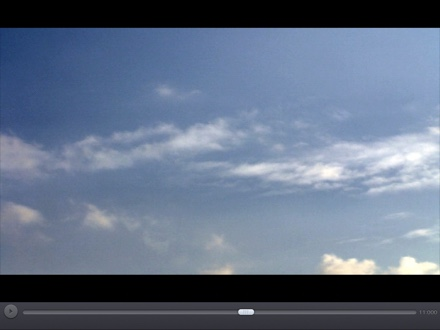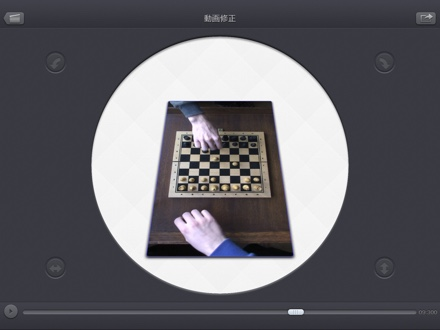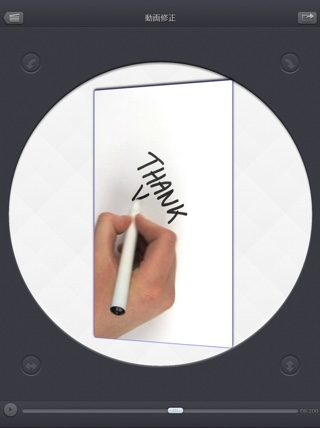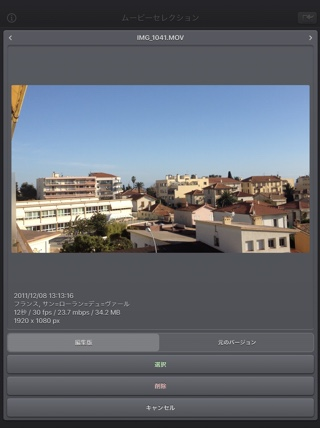# よくある質問

• Q : I'm on iOS8.0 (or higher), and the application does not display most of my videos. Why ?
• Q : The videos in my Camera Roll are not accessible. How to solve that problem?
• Q : The videos imported from my computer are not accessible. How to solve that problem?
• Q : The rotation works on iPhone but not on my computer. What can I do?
• Q : How can I delete videos that have been loaded?
• Q : What video formats can be read?

• 小さな修正と改善。

# 供給